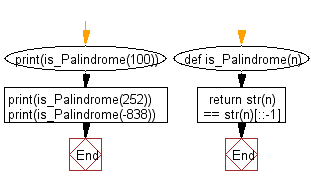﻿ Python: Check whether a given integer is a palindrome or not - w3resource

# Python: Check whether a given integer is a palindrome or not

## Python Basic - 1: Exercise-72 with Solution

Write a Python program to check whether a given integer is a palindrome or not.
Note: An integer is a palindrome when it reads the same backward as forward. Negative numbers are not palindromic.

Sample Solution:

Python Code:

``````def is_Palindrome(n):
return str(n) == str(n)[::-1]
print(is_Palindrome(100))
print(is_Palindrome(252))
print(is_Palindrome(-838))
``````

Sample Output:

```False
True
False
```

Pictorial Presentation:Flowchart:Python Code Editor:

Have another way to solve this solution? Contribute your code (and comments) through Disqus.

What is the difficulty level of this exercise?

Test your Programming skills with w3resource's quiz.

﻿